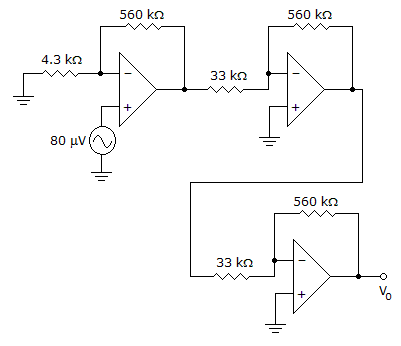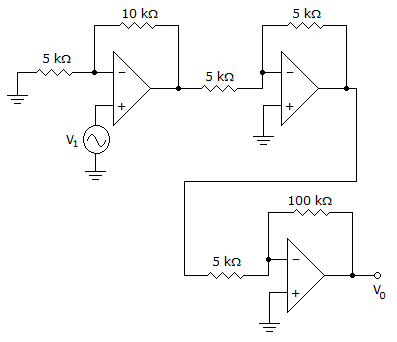# Electronic Devices - Op-Amp Applications

Exercise : Op-Amp Applications - General Questions
21.
Calculate the output voltage.3.02 V
2.03 V
1.78 V
1.50 V
Explanation:
No answer description is available. Let's discuss.

22.
Calculate the output of the second stage op-amp if V1 = 25 mV.–0.075 V
0.525 V
0.06 V
4.2 V
Explanation:
No answer description is available. Let's discuss.

23.
A difference between a passive filter and an active filter is that a passive filter uses amplifier(s), but an active filter does not.
True
False
Explanation:
No answer description is available. Let's discuss.

24.
Calculate the output voltage Vo if V1 = –V2 = 300 mV.0 V
–6 V
6 V
–8 V
Explanation:
No answer description is available. Let's discuss.

25.
Calculate IL for this circuit.3 mA
4 mA
5 mA
6 mA